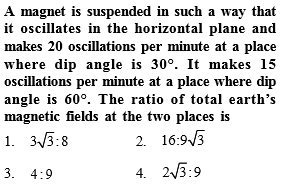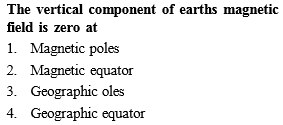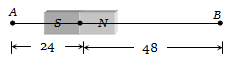NEET Questions Solved

Concept Videos :-

#10 | Earth's Magnetism
#11 | Apparent & True Dip
#12 | Neutral points
#13 | Comparison of Magnetic Field of Earth at Two Points
#14 | Variation of Earth's Magnetic Field

Concept Questions :-

Earth's magnetism
Explanation is a part of a Paid Course. To view Explanation Please buy the course.

Difficulty Level:Concept Videos :-

#10 | Earth's Magnetism
#11 | Apparent & True Dip
#12 | Neutral points
#13 | Comparison of Magnetic Field of Earth at Two Points
#14 | Variation of Earth's Magnetic Field

Concept Questions :-

Earth's magnetism
Explanation is a part of a Paid Course. To view Explanation Please buy the course.

Difficulty Level:

1.

2.

3.

4.  ${\mathrm{tan}}^{-1}\left(\frac{2}{\sqrt{3}}\right)$

Concept Videos :-

#10 | Earth's Magnetism
#11 | Apparent & True Dip
#12 | Neutral points
#13 | Comparison of Magnetic Field of Earth at Two Points
#14 | Variation of Earth's Magnetic Field

Concept Questions :-

Earth's magnetism
Explanation is a part of a Paid Course. To view Explanation Please buy the course.

Difficulty Level:Concept Videos :-

#10 | Earth's Magnetism
#11 | Apparent & True Dip
#12 | Neutral points
#13 | Comparison of Magnetic Field of Earth at Two Points
#14 | Variation of Earth's Magnetic Field

Concept Questions :-

Earth's magnetism
Explanation is a part of a Paid Course. To view Explanation Please buy the course.

Difficulty Level:

A bar magnet of length 3 cm has points A and B along its axis at distances of 24 cm and 48 cm on the opposite sides. Ratio of magnetic fields at these points will be(a) 8                               (b)$\frac{1}{2\sqrt{2}}$

(c) 3                               (d) 4

Concept Videos :-

#10 | Earth's Magnetism
#11 | Apparent & True Dip
#12 | Neutral points
#13 | Comparison of Magnetic Field of Earth at Two Points
#14 | Variation of Earth's Magnetic Field

Concept Questions :-

Earth's magnetism
Explanation is a part of a Paid Course. To view Explanation Please buy the course.

Difficulty Level:

A dip needle in a plane perpendicular to magnetic meridian will remain

(a) Vertical

(b) Horizontal

(c) In any direction

(d) At an angle of dip to the horizontal

Concept Videos :-

#10 | Earth's Magnetism
#11 | Apparent & True Dip
#12 | Neutral points
#13 | Comparison of Magnetic Field of Earth at Two Points
#14 | Variation of Earth's Magnetic Field

Concept Questions :-

Earth's magnetism
Explanation is a part of a Paid Course. To view Explanation Please buy the course.

Difficulty Level:

If the angles of dip at two places are 30o and 45o respectively, then the ratio of horizontal  components of earth's magnetic field at the two places will be

(a) $\sqrt{3}:\sqrt{2}$                       (b) $1:\sqrt{2}$

(c) $1:\sqrt{3}$                          (d) 1:2

Concept Videos :-

#10 | Earth's Magnetism
#11 | Apparent & True Dip
#12 | Neutral points
#13 | Comparison of Magnetic Field of Earth at Two Points
#14 | Variation of Earth's Magnetic Field

Concept Questions :-

Earth's magnetism
Explanation is a part of a Paid Course. To view Explanation Please buy the course.

Difficulty Level:

A line passing through places having zero value of magnetic dip is called

(a) Isoclinic line             (b) Agonic line

(c) Isogonic line             (d) Aclinic line

Concept Videos :-

#10 | Earth's Magnetism
#11 | Apparent & True Dip
#12 | Neutral points
#13 | Comparison of Magnetic Field of Earth at Two Points
#14 | Variation of Earth's Magnetic Field

Concept Questions :-

Earth's magnetism
Explanation is a part of a Paid Course. To view Explanation Please buy the course.

Difficulty Level:

The earth's magnetic field at a certain place has a horizontal component 0.3 Gauss and the total strength 0.5 Gauss. The angle of dip is

(a) ${\mathrm{tan}}^{-1}\left(\frac{3}{4}\right)$                      (b) ${\mathrm{sin}}^{-1}\left(\frac{3}{4}\right)$

(c) ${\mathrm{tan}}^{-1}\left(\frac{4}{3}\right)$                      (d) ${\mathrm{sin}}^{-1}\left(\frac{3}{5}\right)$

Concept Videos :-

#10 | Earth's Magnetism
#11 | Apparent & True Dip
#12 | Neutral points
#13 | Comparison of Magnetic Field of Earth at Two Points
#14 | Variation of Earth's Magnetic Field

Concept Questions :-

Earth's magnetism
Explanation is a part of a Paid Course. To view Explanation Please buy the course.

Difficulty Level:

At a certain place, the horizontal component B0 and the vertical component V0 of the earth's magnetic field are equal in magnitude. The total intensity at the place will be

(a) ${B}_{0}$                            (b) ${B}_{0}^{2}$

(c) $2{B}_{0}$                          (d) $\sqrt{2}{B}_{0}$

Concept Videos :-

#10 | Earth's Magnetism
#11 | Apparent & True Dip
#12 | Neutral points
#13 | Comparison of Magnetic Field of Earth at Two Points
#14 | Variation of Earth's Magnetic Field

Concept Questions :-

Earth's magnetism
Explanation is a part of a Paid Course. To view Explanation Please buy the course.

Difficulty Level: